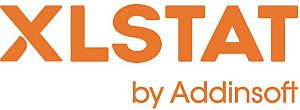# XLSTAT - One sample t and z tests

## Principle of the one-sample t- and z-tests

This tool is used to compare the average of a sample represented by µ with a reference value.

## When to use the Student's t-test or the z-test

Two parametric tests are possible but they should be used on certain conditions:

### The Student's t-test

Use the Student's t-test when the true variance of the population from which the sample has been extracted is not known; the variance of sample s² is used as variance estimator.

### The z-test

Use the z-test when the true variance σ² of the population is known.

## The Student's t-test and the z-test are parametric tests

Both the Student's t-test and the z-test are said to be parametric as their use requires the assumption that the samples are distributed normally. Moreover, it also assumed that the observations are independent and identically distributed.

## Two-tailed or one-tailed test

Three types of test are possible depending on the alternative hypothesis chosen:

• For the two-tailed test, the null H0 and alternative Ha hypotheses are as follows:
H0 : µ = µ0
Ha : µ ≠ µ0
• In the left one-tailed test, the following hypotheses are used:
H0 : µ = µ0
Ha : µ < µ0
• In the right one-tailed test, the following hypotheses are used:
H0 : µ = µ0
Ha : µ > µ0This analysis is available in the XLStat-Basic addin for Microsoft ExcelKovach Computing Services (KCS) was founded in 1993 by Dr. Warren Kovach. The company specializes in the development and marketing of inexpensive and easy-to-use statistical software for scientists, as well as in data analysis consulting.

### Get in Touch

• Email:
sales@kovcomp.com